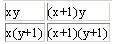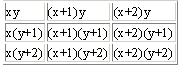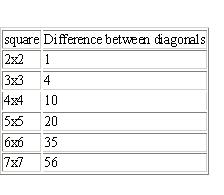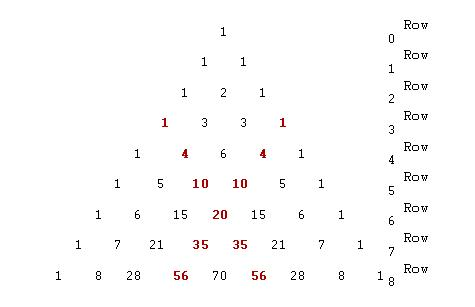#### You may also like### Doodles

Draw a 'doodle' - a closed intersecting curve drawn without taking pencil from paper. What can you prove about the intersections?### Russian Cubes

I want some cubes painted with three blue faces and three red faces. How many different cubes can be painted like that?### Picture Story

Can you see how this picture illustrates the formula for the sum of the first six cube numbers?

# Multiplication Square

##### Age 14 to 16Challenge Level

Well done to Maulik aged 11 who sent in some nice work on this problem. Neil's solution is given below.

For a 2 by 2 square with column headings of x and x+1, and row headings of y and y+1, Neil says that:

For the two by two square you can always express it algebraically like this:So the diagonal from top right to bottom left is:

$(x+1)y+x(y+1) = xy+y+xy+x = 2xy+x+y$

Lets call that Z.

The diagonal from top left to bottom right is:

$xy+(x+1)(y+1) = xy+xy+x+y+1 = 2xy+x+y+1$

So the first diagonal is Z and the second Z+1 so the diagonal from top left to bottom right is always 1 more than the diagonal from top right to bottom left.

For a 3 by 3 square with column headings of x, x+1 and x+2, and row headings of y, y+1 and y+2, Neil says that:

The 3 by 3 square looks like this:The diagonal from top right to bottom left is:

$\begin{split}x(y+2)+(x+1)(y+1)+(x+2)y &= xy+2x+xy+x+y+1+xy+2y \\&= 3xy+3x+3y+1\end{split}$

The diagonal from top left to bottom right is:

$\begin{split}xy+(x+1)(y+1)+(x+2)(y+2) &= xy+xy+x+y+1+xy+2x+2y+4 \\&= 3xy+3x+3y+5 \end{split}$

Let's say that $3xy+3x+3y = W$

The diagonal from top right to bottom left is W+1.

The diagonal from top left to bottom right is W+5. So the difference between the diagonals is 4.

More generally

Continuing with the same method:Note that
1 = 1 ²
4 = 2 ²
10 = 1 ² + 3 ²
20 = 2 ² + 4 ²
35 =1 ² + 3 ² + 5 ²
56 = 2 ² + 4 ² + 6 ²
84 = 1 ² + 3 ² + 5 ² + 7 ²

Neil goes on to point out that the differences are the tetrahedral numbers.
Tetrahedral numbers are the sum of consecutive triangular numbers.
The formula is 1/6n(n+1)(n+2).
The first few tetrahedral numbers are 1, 4, 10, 20, 35, 56, 84, 120, ...
The tetrahedral numbers are found in the fourth diagonal of Pascal's triangle: